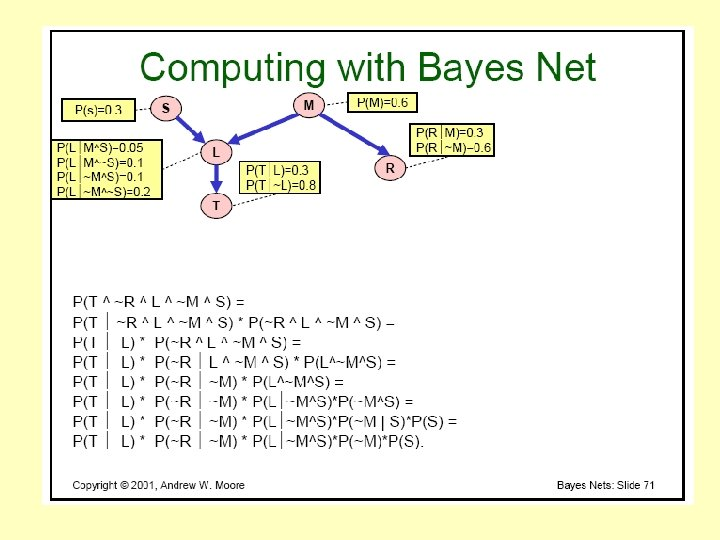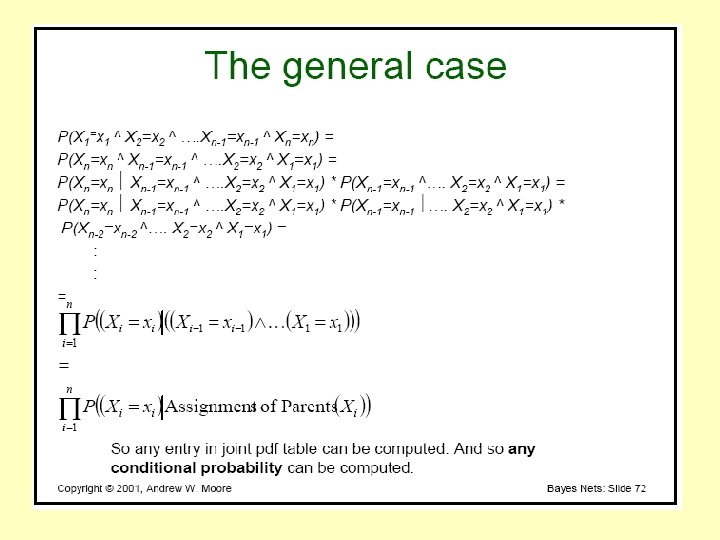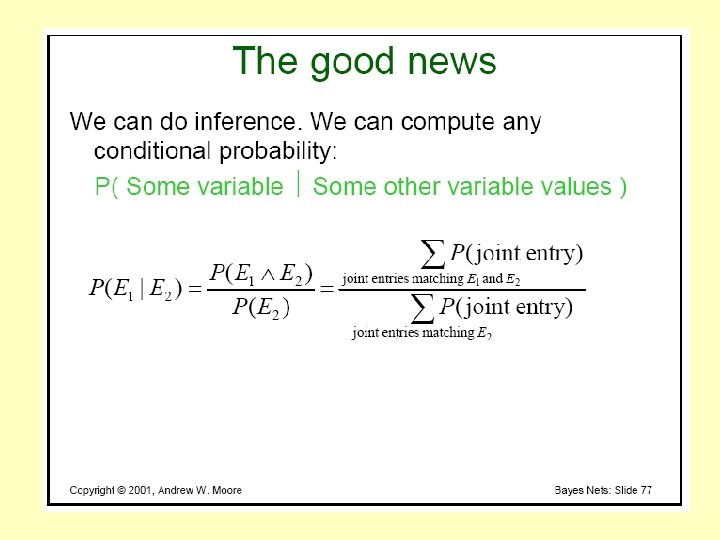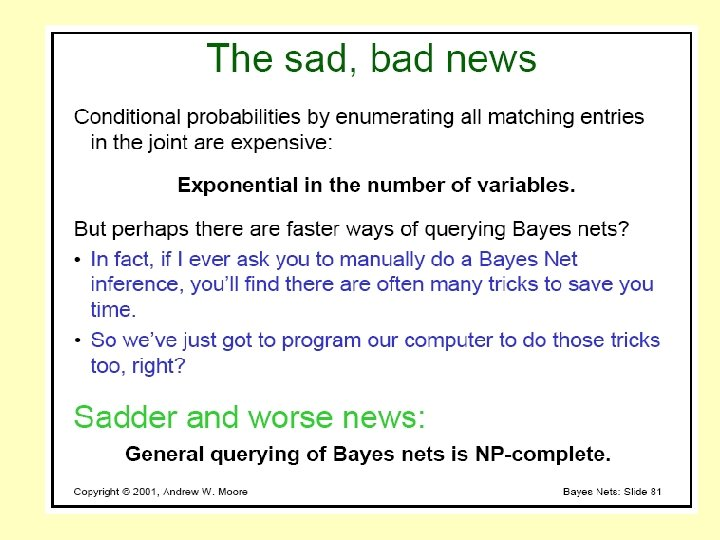# CS 4100 Artificial Intelligence Prof C Hafner Class

• Slides: 81
Download presentationCS 4100 Artificial Intelligence Prof. C. Hafner Class Notes March 15 and 20, 2012Outline • Midterm planning problem: solution http: //www. ccs. neu. edu/course/cs 4100 sp 12/classnotes/midterm-planning. doc • Discuss term projects • Continue uncertain reasoning in AI – Probability distribution (review) – Conditional Probability and the Chain Rule (cont. ) – Bayes’ Rule – Independence, “Expert” systems and the combinatorics of joint probabilities – Bayes networks – Assignment 6Term Projects – The Process 1. Form teams of 3 or 4 people – 10 -12 teams 2. Before next class (Mar 20) each team send an email a. Name and a main contact person (email) b. All team members’ names and email addresses c. You can reserve a topic asap (first request) 3. Brief written project proposal due Fri March 23 10 pm (email) 4. Each team will a. b. c. submit a written project report (due April 17, last day of class) a running computer application (due April 17, last day of class) make a presentation of 15 minutes on their project (April 12 & 17) 5. Attendance is required and will be taken on April 12 & 17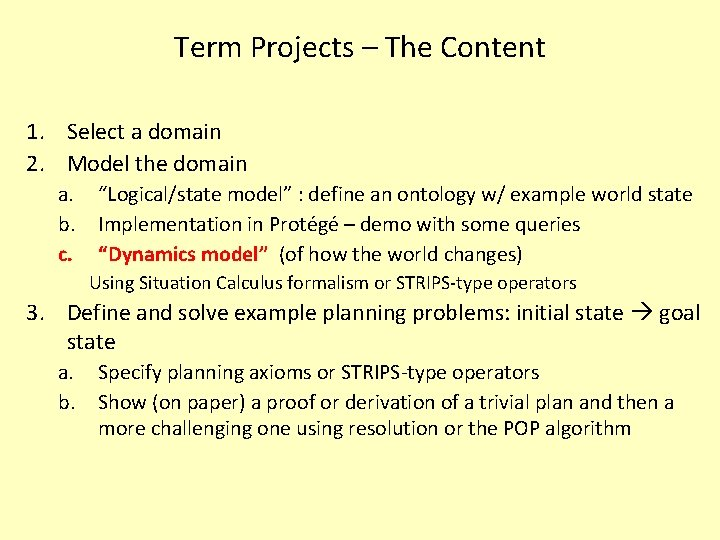Term Projects – The Content 1. Select a domain 2. Model the domain a. b. c. “Logical/state model” : define an ontology w/ example world state Implementation in Protégé – demo with some queries “Dynamics model” (of how the world changes) Using Situation Calculus formalism or STRIPS-type operators 3. Define and solve example planning problems: initial state goal state a. b. Specify planning axioms or STRIPS-type operators Show (on paper) a proof or derivation of a trivial plan and then a more challenging one using resolution or the POP algorithmTerm Projects – Choosing Domains Travel domains: Boston T, other kinds of trips or vacations Cooking domains: planning a meal, a dinner party, preparing a recipe Sports domains: One league or tournament? Gaming domains: model a game that requires some strategy Military mission planning Exercise session/program planning (including use of equipment) Making a movie An issue is granularity: how fine a level of detail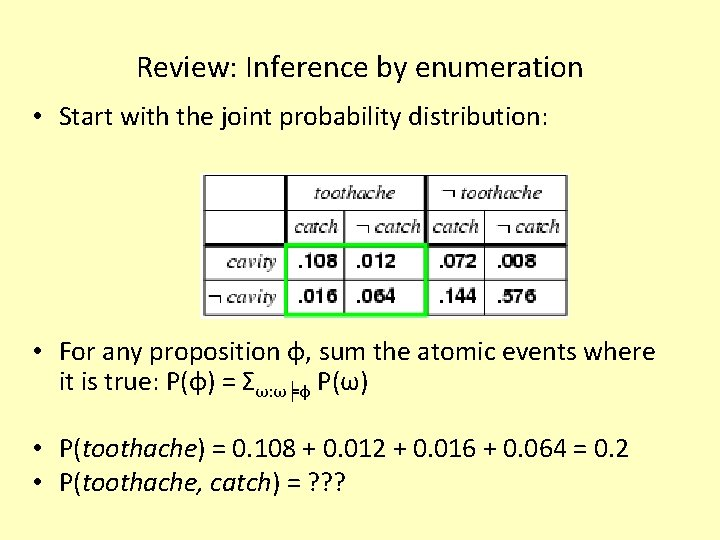Review: Inference by enumeration • Start with the joint probability distribution: • For any proposition φ, sum the atomic events where it is true: P(φ) = Σω: ω╞φ P(ω) • P(toothache) = 0. 108 + 0. 012 + 0. 016 + 0. 064 = 0. 2 • P(toothache, catch) = ? ? ?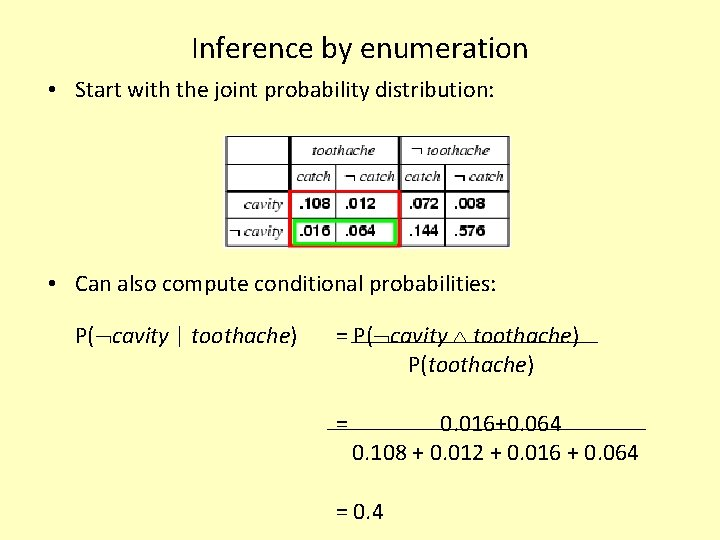Inference by enumeration • Start with the joint probability distribution: • Can also compute conditional probabilities: P( cavity | toothache) = P( cavity toothache) P(toothache) = 0. 016+0. 064 0. 108 + 0. 012 + 0. 016 + 0. 064 = 0. 4Conditional probability and Bayes Rule • Definition of conditional probability: P(a | b) = P(a b) / P(b) if P(b) > 0 • Product rule gives an alternative formulation: P(a b) = P(a | b) P(b) = P(b | a) P(a) • Combine these to derive: Bayes' rule: P(a | b) = P(b | a) P(a) / P(b) • Useful for assessing diagnostic probability from causal probability: – P(Cause|Effect) = P(Effect|Cause) P(Cause) / P(Effect) – E. g. , let M be meningitis, S be stiff neck: P(m|s) = P(s|m) P(m) / P(s) = 0. 8 × 0. 0001 / 0. 1 = 0. 0008 – Note: posterior probability of meningitis still very small!The Chain Rule • Chain rule is derived by successive application of product rule: P(X 1, …, Xn) = P(X 1, . . . , Xn-1) P(Xn | X 1, . . . , Xn-1) = P(X 1, . . . , Xn-2) P(Xn-1 | X 1, . . . , Xn-2) P(Xn | X 1, . . . , Xn-1) =… = P(X 1) P(X 2 | X 1) P(X 3 | X 1, X 2). . . P(Xn | X 1, . . . , Xn-1) OR: πi= 1 to n P(Xi | X 1, … , Xi-1)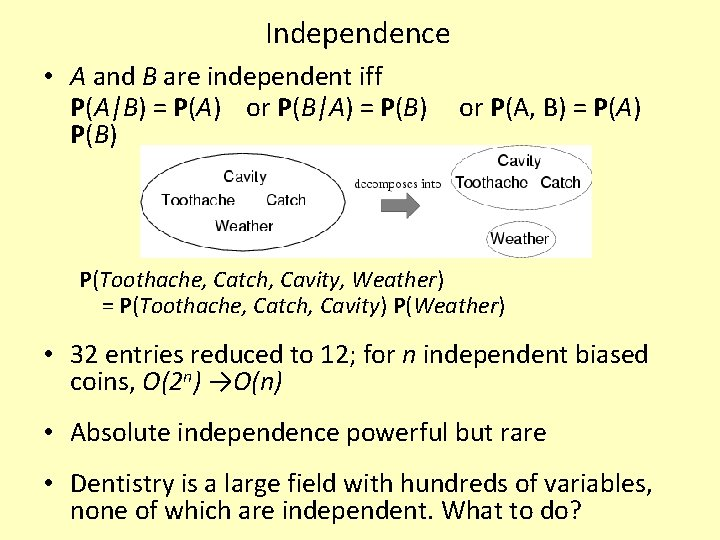Independence • A and B are independent iff P(A|B) = P(A) or P(B|A) = P(B) or P(A, B) = P(A) P(Toothache, Catch, Cavity, Weather) = P(Toothache, Catch, Cavity) P(Weather) • 32 entries reduced to 12; for n independent biased coins, O(2 n) →O(n) • Absolute independence powerful but rare • Dentistry is a large field with hundreds of variables, none of which are independent. What to do?Example: Expert Systems for Medical Diagnosis • 100 diseases (assume only one at a time!) • 20 symptoms • # of parameters needed to calculate P(Di) when a patient provides his/her symptoms • Strategy to reduce the size: assume independence of all symptoms • Recalculate number of parameters needed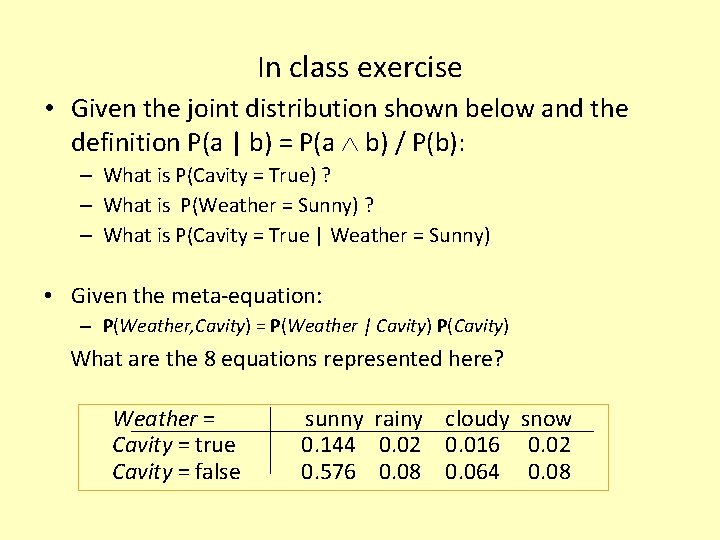In class exercise • Given the joint distribution shown below and the definition P(a | b) = P(a b) / P(b): – What is P(Cavity = True) ? – What is P(Weather = Sunny) ? – What is P(Cavity = True | Weather = Sunny) • Given the meta-equation: – P(Weather, Cavity) = P(Weather | Cavity) P(Cavity) What are the 8 equations represented here? Weather = Cavity = true Cavity = false sunny rainy cloudy snow 0. 144 0. 02 0. 016 0. 02 0. 576 0. 08 0. 064 0. 08Bayes' Rule and conditional independence P(Cavity | toothache catch) = αP(toothache catch | Cavity) P(Cavity) = αP(toothache | Cavity) P(catch | Cavity) P(Cavity) • This is an example of a naïve Bayes model: P(Cause, Effect 1, … , Effectn) = P(Cause) πi. P(Effecti|Cause) • Total number of parameters is linear in nConditional independence • P(Toothache, Cavity, Catch) has 23 – 1 = 7 independent entries • If I have a cavity, the probability that the probe catches in it doesn't depend on whether I have a toothache: (1) P(catch | toothache, cavity) = P(catch | cavity) • The same independence holds if I haven't got a cavity: (2) P(catch | toothache, cavity) = P(catch | cavity) • Catch is conditionally independent of Toothache given Cavity: P(Catch | Toothache, Cavity) = P(Catch | Cavity) • Equivalent statements: P(Toothache | Catch, Cavity) = P(Toothache | Cavity) P(Toothache, Catch | Cavity) = P(Toothache | Cavity) P(Catch | Cavity)Bayesian networks • A simple, graphical notation for conditional independence assertions and hence for compact specification of full joint distributions • Syntax: – a set of nodes, one per variable – a directed, acyclic graph (link ≈ "directly influences") – a conditional distribution for each node given its parents: P (Xi | Parents (Xi)) • In the simplest case, conditional distribution represented as a conditional probability table (CPT) giving the distribution over Xi for each combination of parent values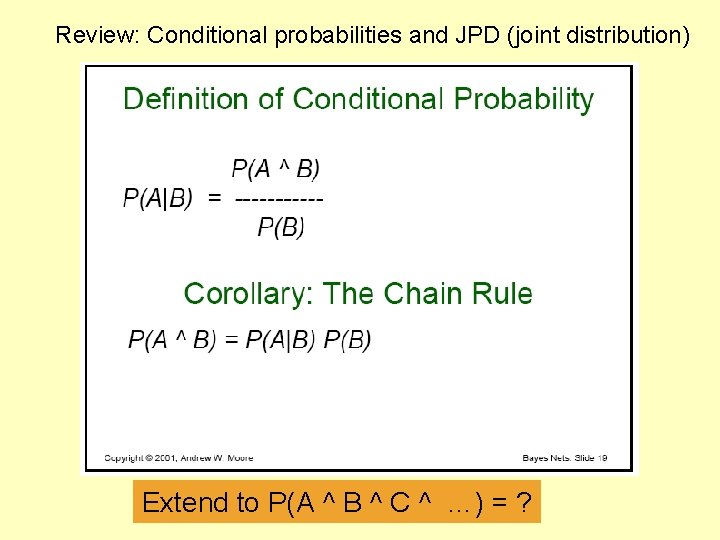Review: Conditional probabilities and JPD (joint distribution) Extend to P(A ^ B ^ C ^ …) = ?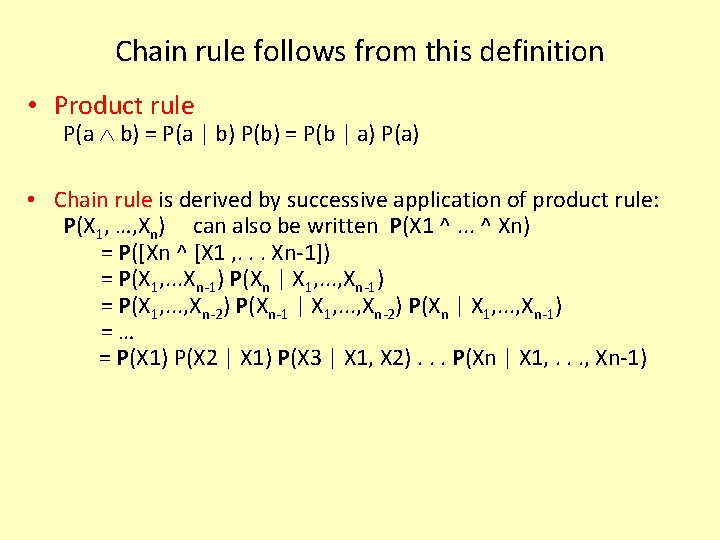Chain rule follows from this definition • Product rule P(a b) = P(a | b) P(b) = P(b | a) P(a) • Chain rule is derived by successive application of product rule: P(X 1, …, Xn) can also be written P(X 1 ^. . . ^ Xn) = P([Xn ^ [X 1 , . . . Xn-1]) = P(X 1, . . . Xn-1) P(Xn | X 1, . . . , Xn-1) = P(X 1, . . . , Xn-2) P(Xn-1 | X 1, . . . , Xn-2) P(Xn | X 1, . . . , Xn-1) =… = P(X 1) P(X 2 | X 1) P(X 3 | X 1, X 2). . . P(Xn | X 1, . . . , Xn-1)Conditional Prob. example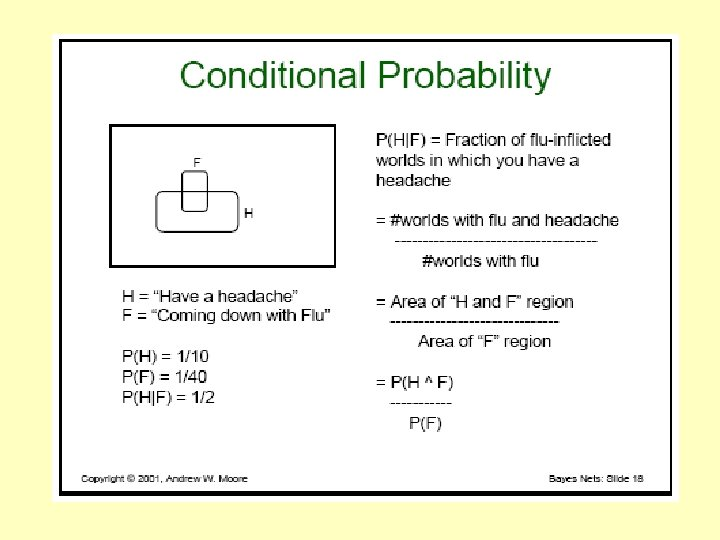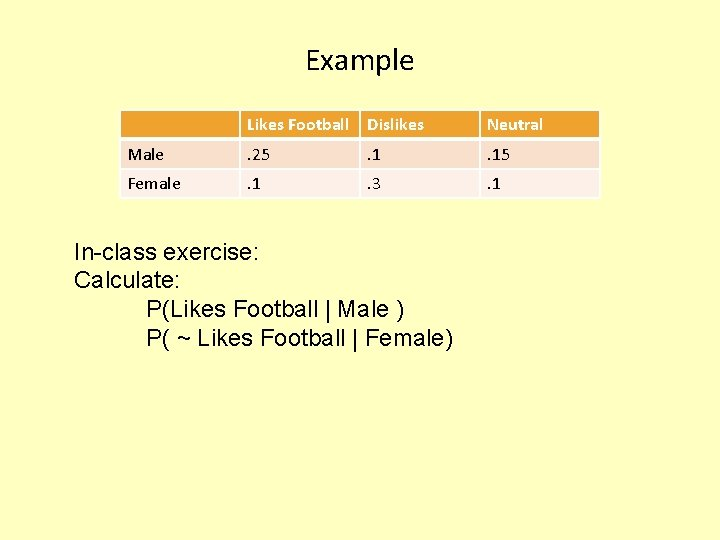Example Likes Football Dislikes Neutral Male . 25 . 15 Female . 1 . 3 . 1 In-class exercise: Calculate: P(Likes Football | Male ) P( ~ Likes Football | Female)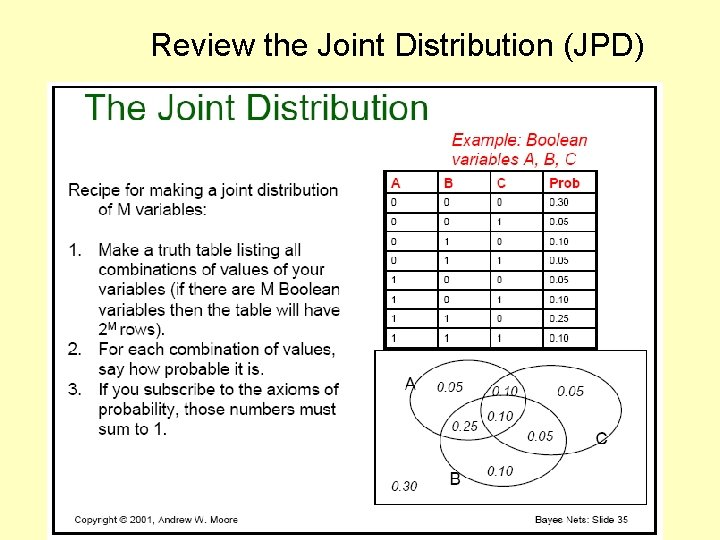Review the Joint Distribution (JPD)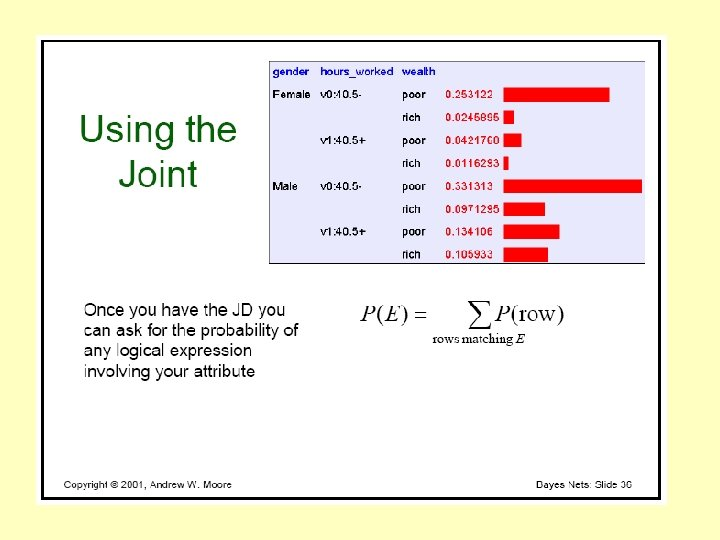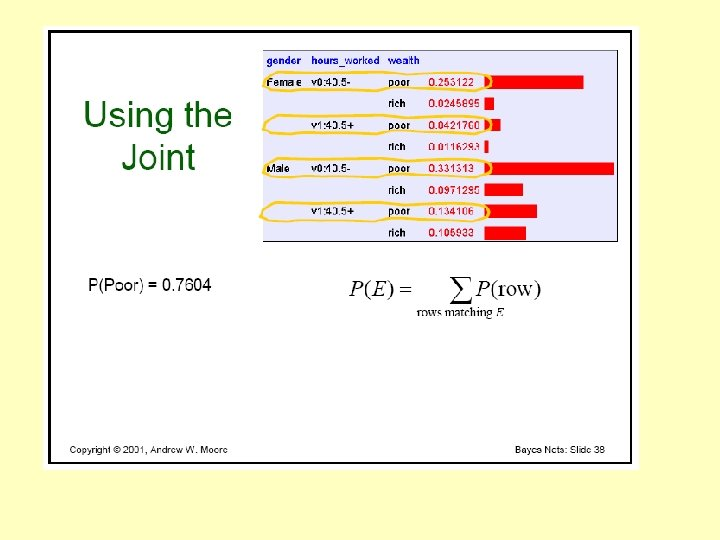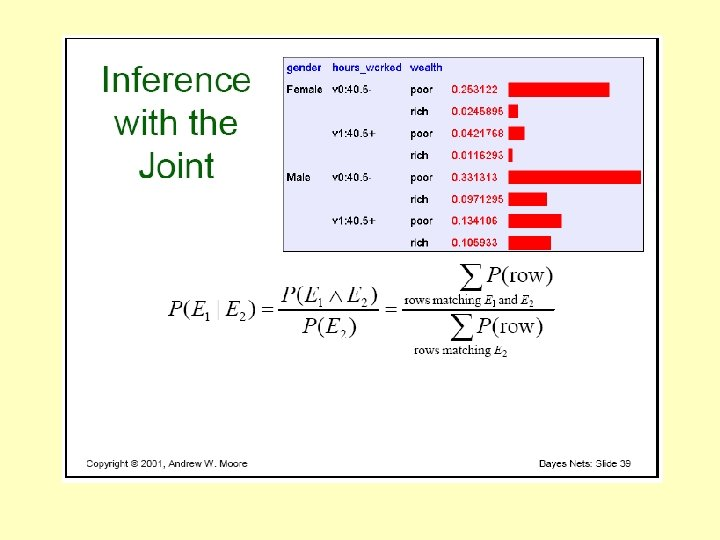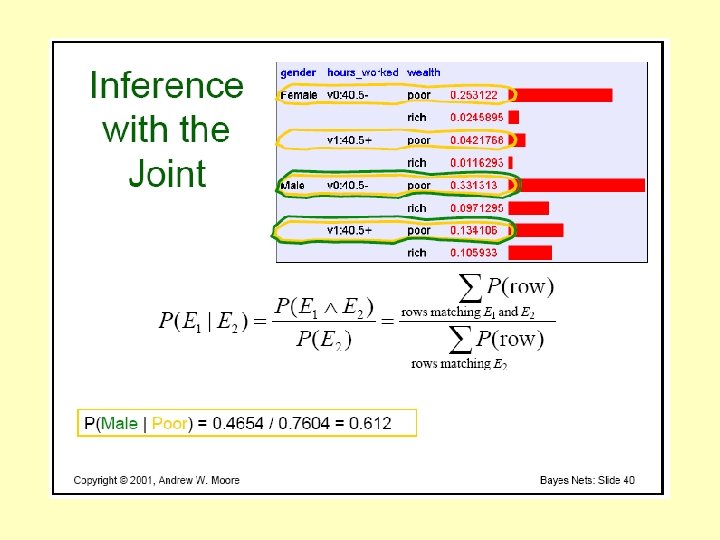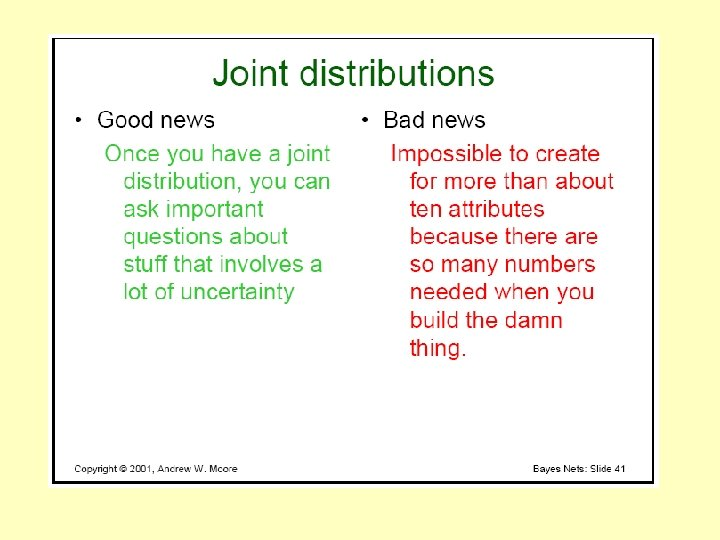What assumption can we make ?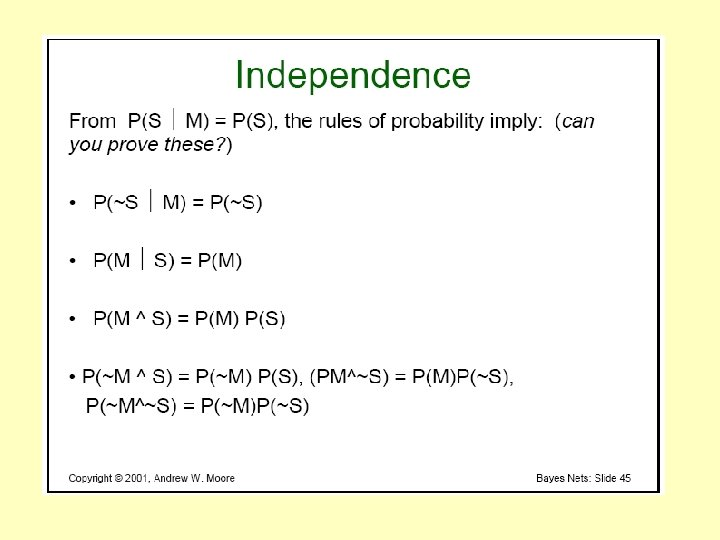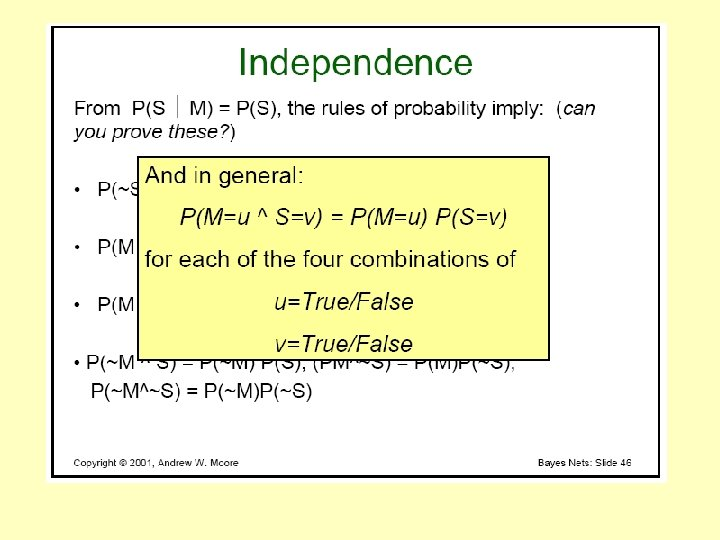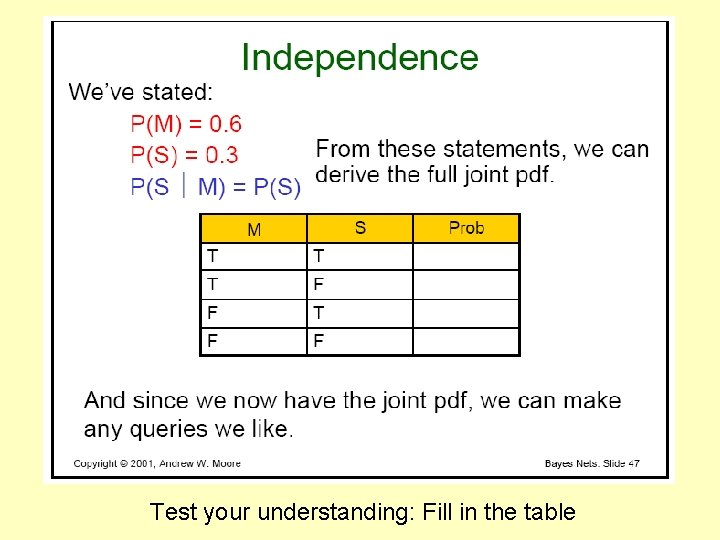Test your understanding: Fill in the tableStructure for CP-based AI Models Given a set of RV’s X, typically, we are interested in the posterior joint distribution of the query variables Y given specific values e for the evidence variables E Let the hidden variables be H = X - Y – E Then the required calculation of P(Y | E) is done by summing out the hidden variables: P( Y | E = e) = αP(Y ^ E = e) or αΣh. P(Y ^ E= e ^ H = h) Note: what is α ? Given the definition: P(a | b) = P(a b) / P(b) α is the denominator 1/P(E=e) can be calculated from the joint distribution as: Σh. P(E= e ^ H = h)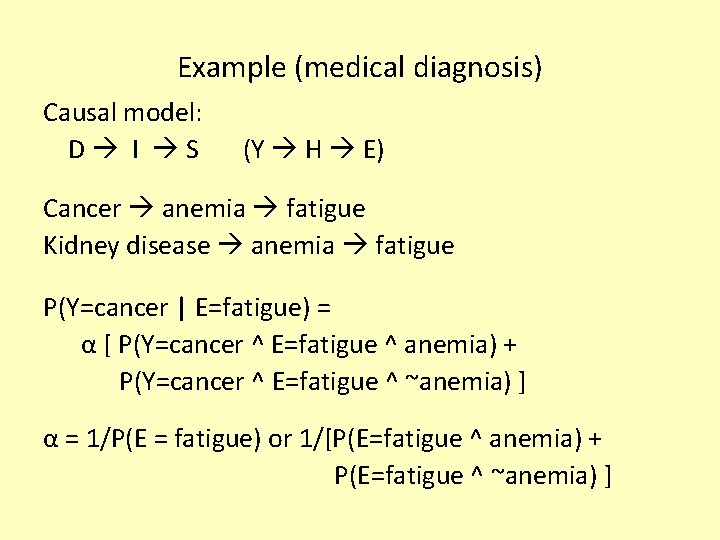Example (medical diagnosis) Causal model: D I S (Y H E) Cancer anemia fatigue Kidney disease anemia fatigue P(Y=cancer | E=fatigue) = α [ P(Y=cancer ^ E=fatigue ^ anemia) + P(Y=cancer ^ E=fatigue ^ ~anemia) ] α = 1/P(E = fatigue) or 1/[P(E=fatigue ^ anemia) + P(E=fatigue ^ ~anemia) ]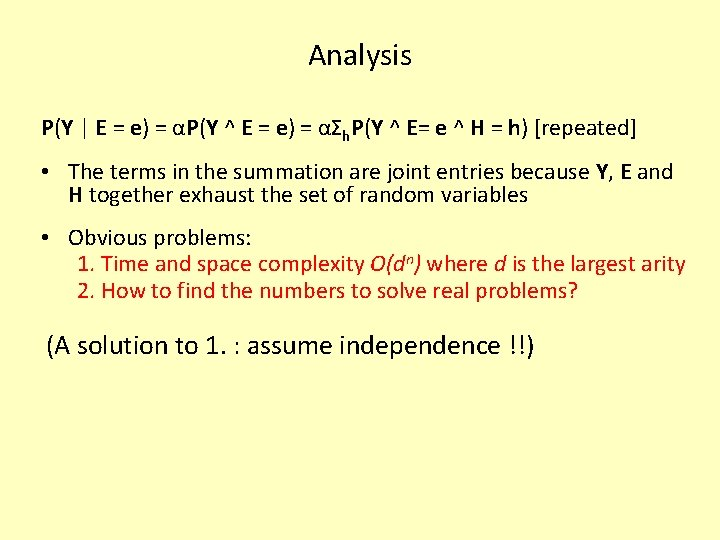Analysis P(Y | E = e) = αP(Y ^ E = e) = αΣh. P(Y ^ E= e ^ H = h) [repeated] • The terms in the summation are joint entries because Y, E and H together exhaust the set of random variables • Obvious problems: 1. Time and space complexity O(dn) where d is the largest arity 2. How to find the numbers to solve real problems? (A solution to 1. : assume independence !!)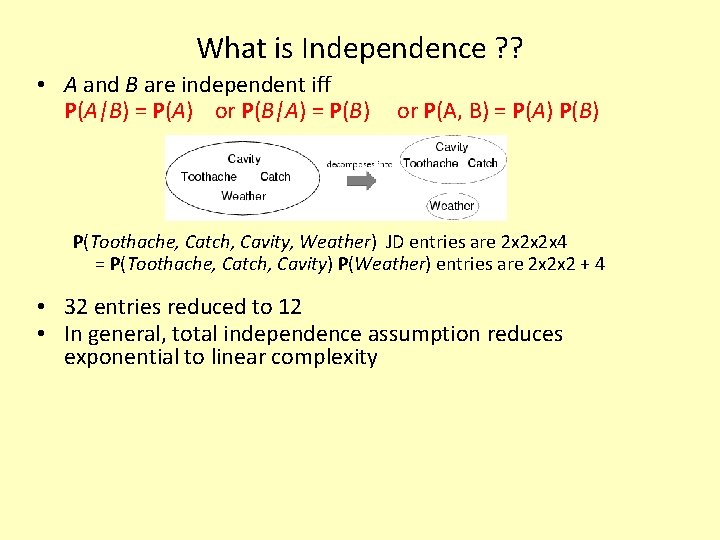What is Independence ? ? • A and B are independent iff P(A|B) = P(A) or P(B|A) = P(B) or P(A, B) = P(A) P(B) P(Toothache, Catch, Cavity, Weather) JD entries are 2 x 2 x 2 x 4 = P(Toothache, Catch, Cavity) P(Weather) entries are 2 x 2 x 2 + 4 • 32 entries reduced to 12 • In general, total independence assumption reduces exponential to linear complexity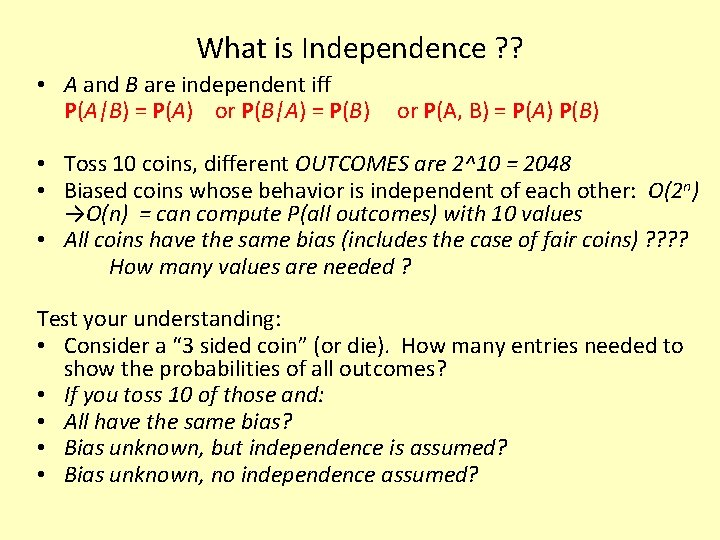What is Independence ? ? • A and B are independent iff P(A|B) = P(A) or P(B|A) = P(B) or P(A, B) = P(A) P(B) • Toss 10 coins, different OUTCOMES are 2^10 = 2048 • Biased coins whose behavior is independent of each other: O(2 n) →O(n) = can compute P(all outcomes) with 10 values • All coins have the same bias (includes the case of fair coins) ? ? How many values are needed ? Test your understanding: • Consider a “ 3 sided coin” (or die). How many entries needed to show the probabilities of all outcomes? • If you toss 10 of those and: • All have the same bias? • Bias unknown, but independence is assumed? • Bias unknown, no independence assumed?Example: Expert Systems for Medical Diagnosis • 10 diseases • 20 symptoms • # of parameters needed to calculate P(D | S) for all combinations using a JPD • Strategy to reduce the size of the model: assume mutual independence of symptoms and diseases Recalculate number of parameters needed • Absolute independence powerful but rare • Medicine is a large field with hundreds of variables, many of which are not independent. What to do?Problem 2: We still need to find the numbers Assuming independence, doctors may be able to estimate: P(symptom | disease) for each S/D pair (causal reasoning) While what we need to know s/he may not be able to estimate as easily: P(disease | symptom) Thus, the importance of Bayes rule in probabilistic AI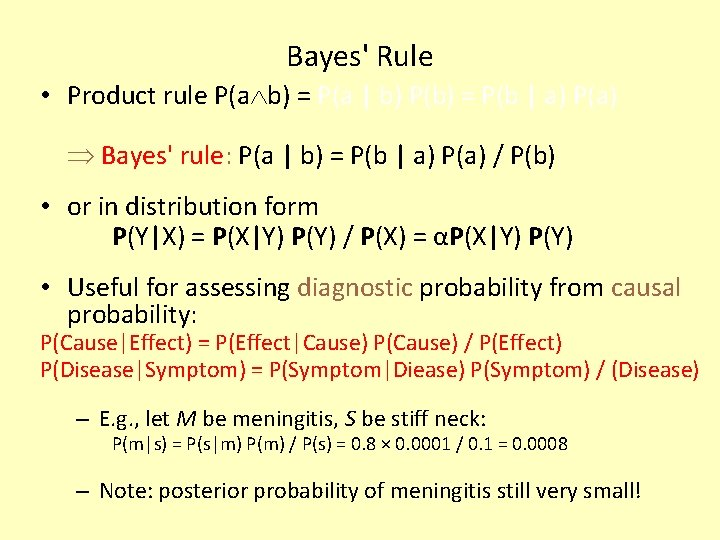Bayes' Rule • Product rule P(a b) = P(a | b) P(b) = P(b | a) P(a) Bayes' rule: P(a | b) = P(b | a) P(a) / P(b) • or in distribution form P(Y|X) = P(X|Y) P(Y) / P(X) = αP(X|Y) P(Y) • Useful for assessing diagnostic probability from causal probability: P(Cause|Effect) = P(Effect|Cause) P(Cause) / P(Effect) P(Disease|Symptom) = P(Symptom|Diease) P(Symptom) / (Disease) – E. g. , let M be meningitis, S be stiff neck: P(m|s) = P(s|m) P(m) / P(s) = 0. 8 × 0. 0001 / 0. 1 = 0. 0008 – Note: posterior probability of meningitis still very small!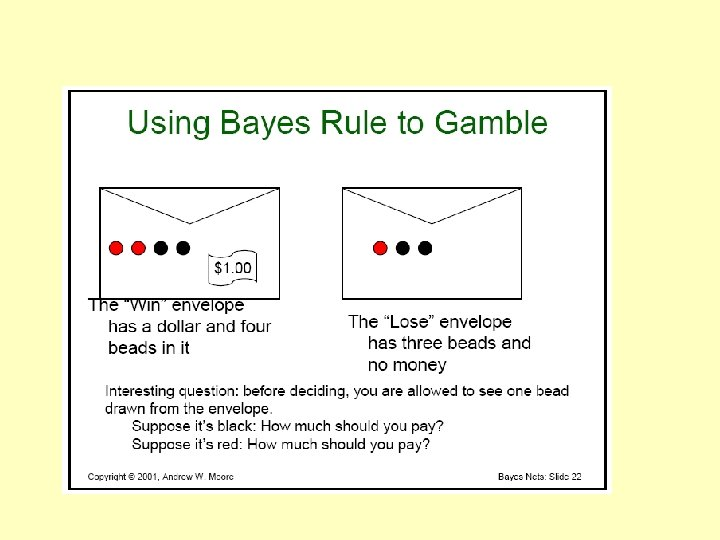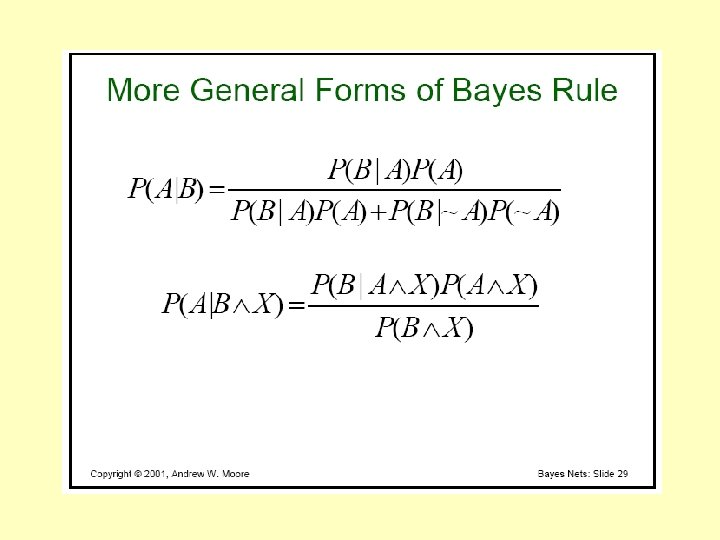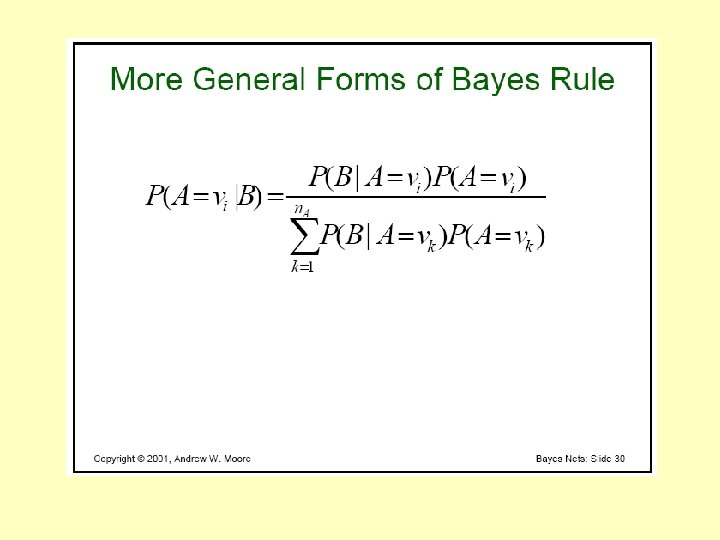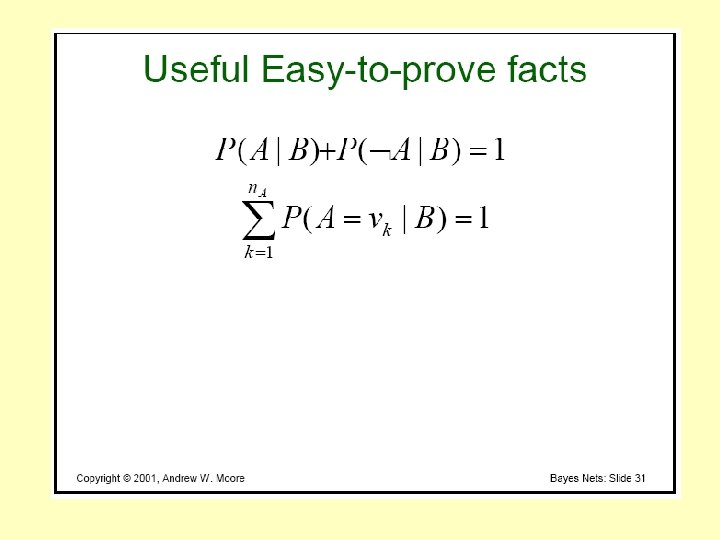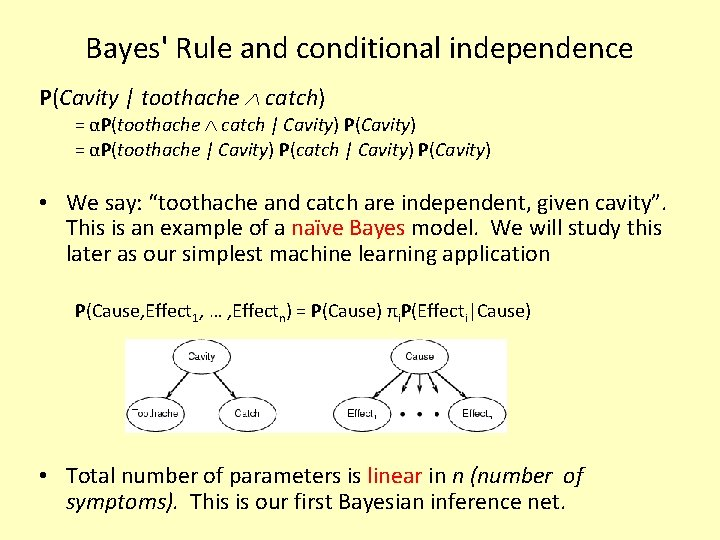Bayes' Rule and conditional independence P(Cavity | toothache catch) = αP(toothache catch | Cavity) P(Cavity) = αP(toothache | Cavity) P(catch | Cavity) P(Cavity) • We say: “toothache and catch are independent, given cavity”. This is an example of a naïve Bayes model. We will study this later as our simplest machine learning application P(Cause, Effect 1, … , Effectn) = P(Cause) πi. P(Effecti|Cause) • Total number of parameters is linear in n (number of symptoms). This is our first Bayesian inference net.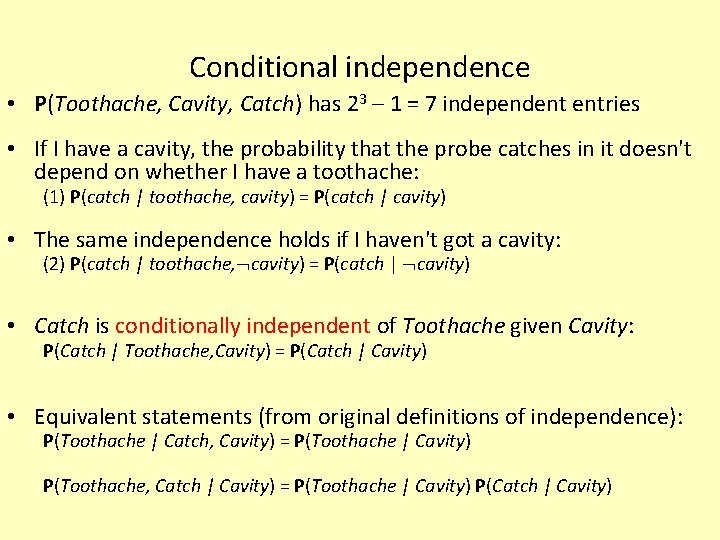Conditional independence • P(Toothache, Cavity, Catch) has 23 – 1 = 7 independent entries • If I have a cavity, the probability that the probe catches in it doesn't depend on whether I have a toothache: (1) P(catch | toothache, cavity) = P(catch | cavity) • The same independence holds if I haven't got a cavity: (2) P(catch | toothache, cavity) = P(catch | cavity) • Catch is conditionally independent of Toothache given Cavity: P(Catch | Toothache, Cavity) = P(Catch | Cavity) • Equivalent statements (from original definitions of independence): P(Toothache | Catch, Cavity) = P(Toothache | Cavity) P(Toothache, Catch | Cavity) = P(Toothache | Cavity) P(Catch | Cavity)Conditional independence contd. • Write out full joint distribution using chain rule: P(Toothache, Catch, Cavity) = P(Toothache | Catch, Cavity) P(Catch | Cavity) P(Cavity) = P(Toothache | Cavity) P(Catch | Cavity) P(Cavity) I. e. , 2 + 1 = 5 independent numbers • In most cases, the use of conditional independence reduces the size of the representation of the joint distribution from exponential in n to linear in n. • Conditional independence is our most basic and robust form of knowledge about uncertain environments.Remember this examples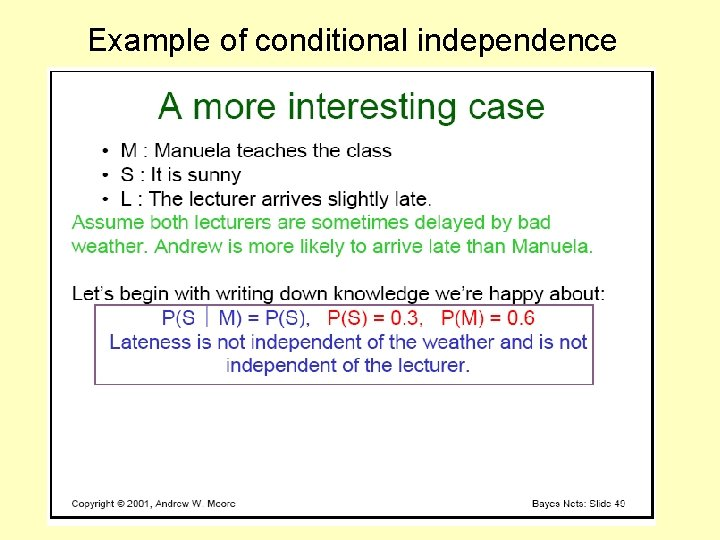Example of conditional independence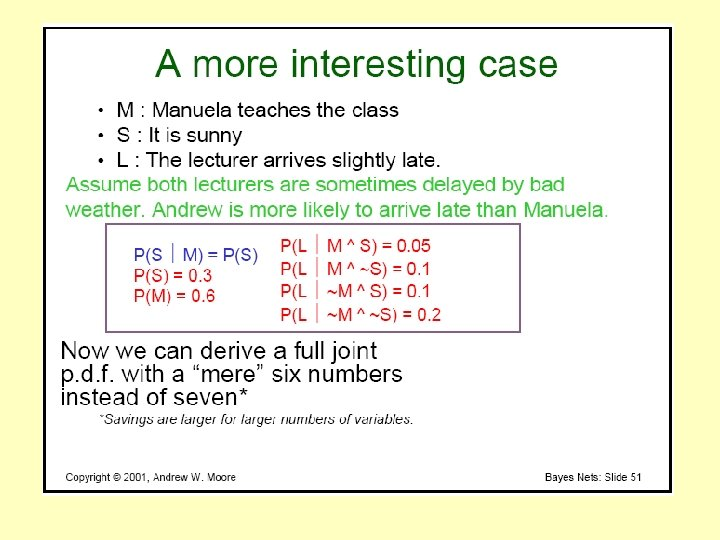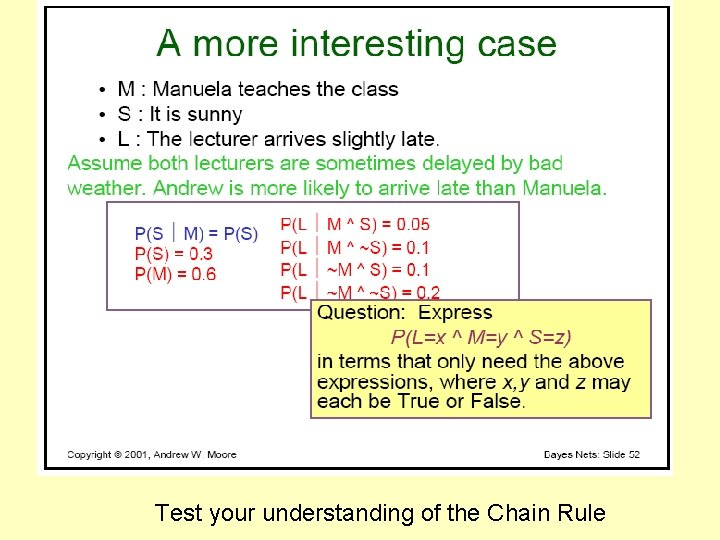Test your understanding of the Chain Rule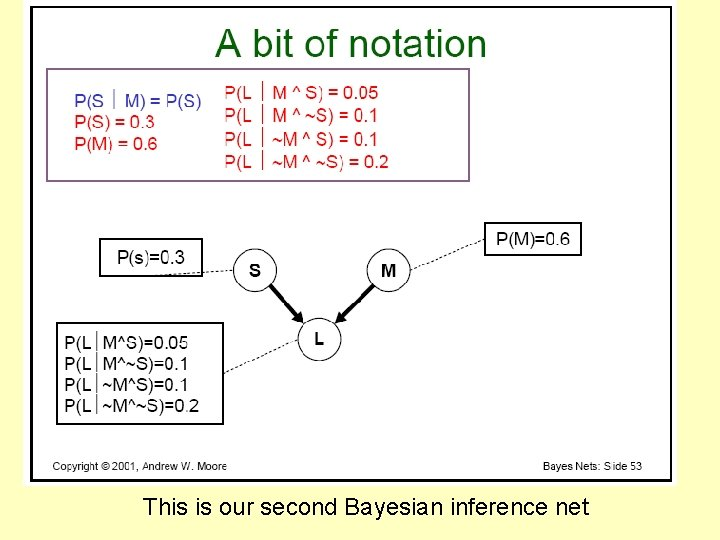This is our second Bayesian inference net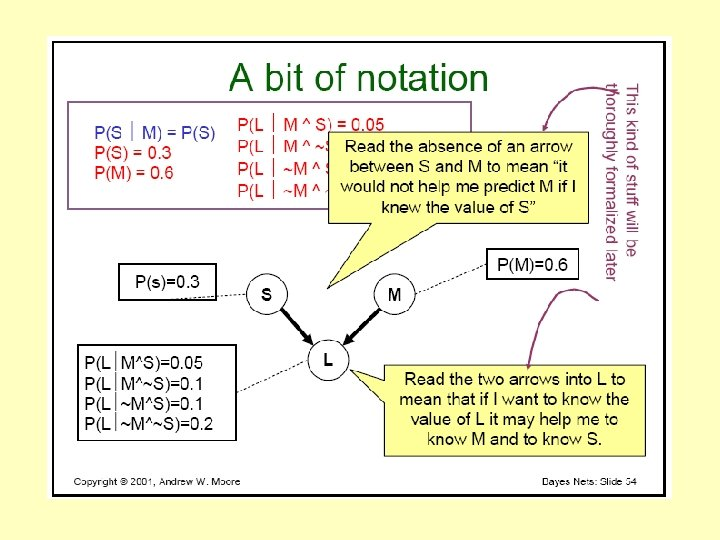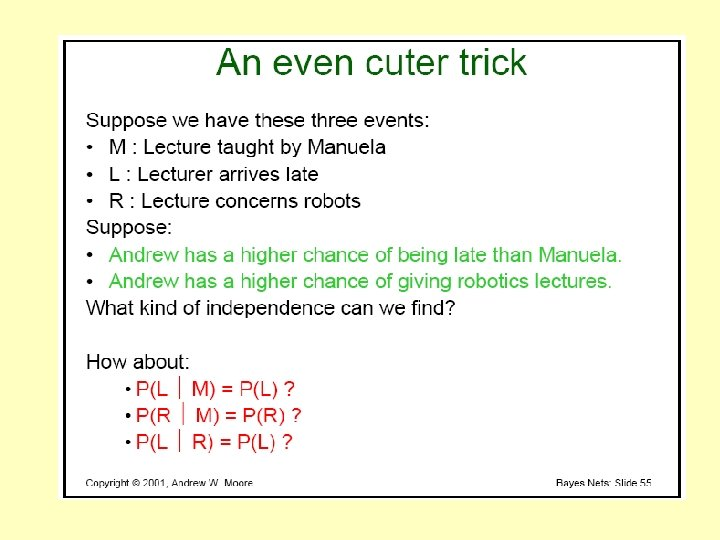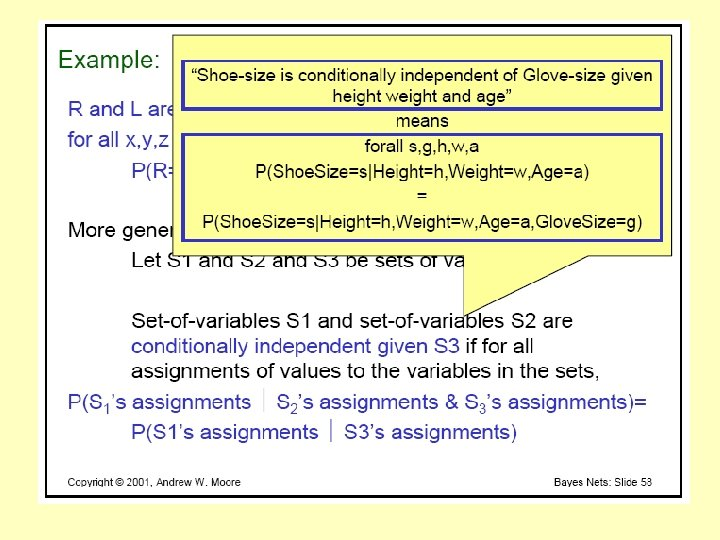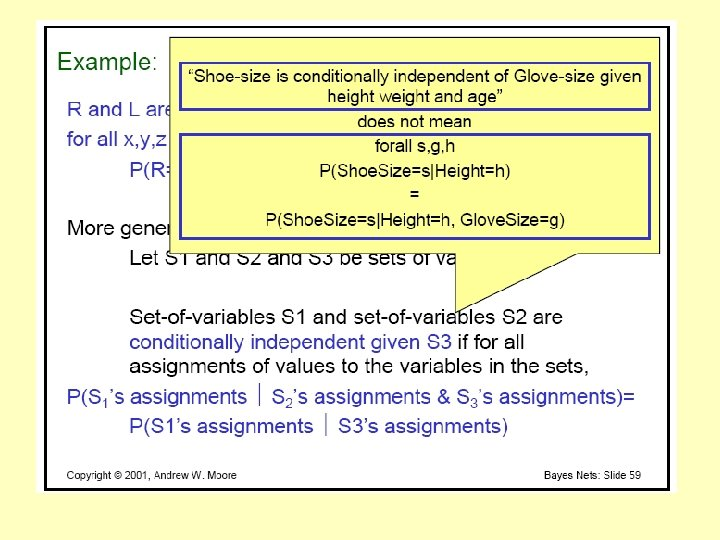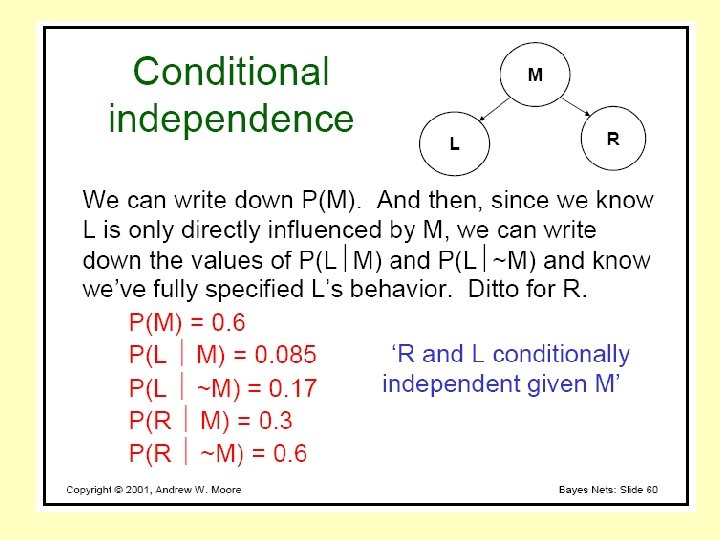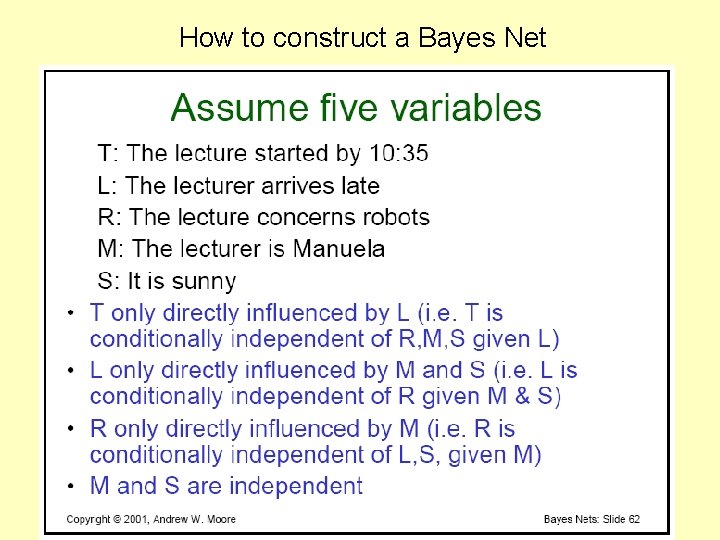How to construct a Bayes Net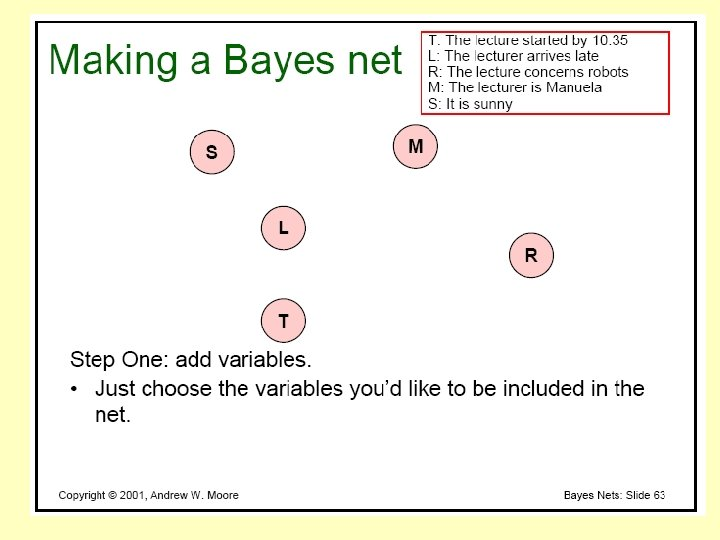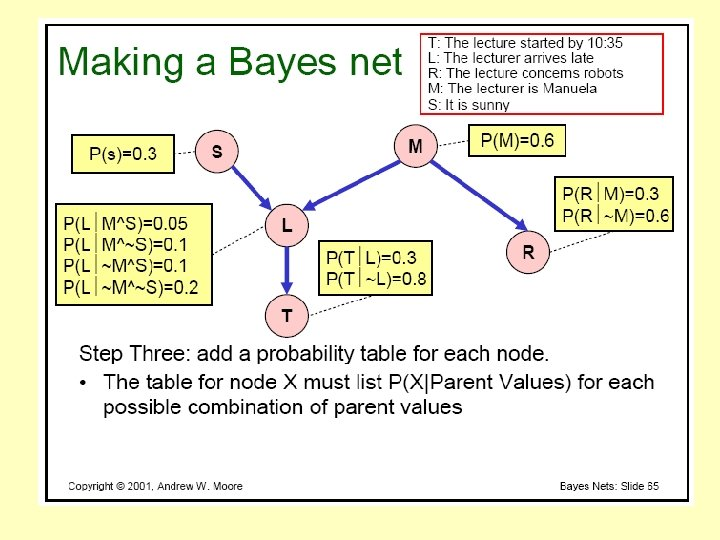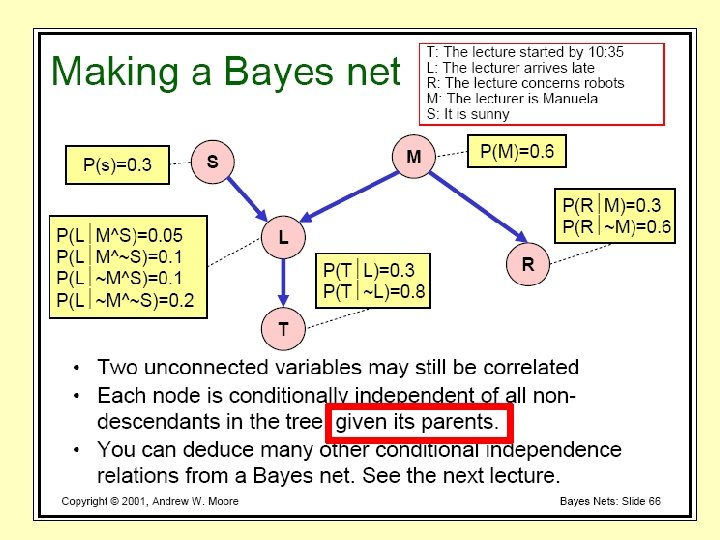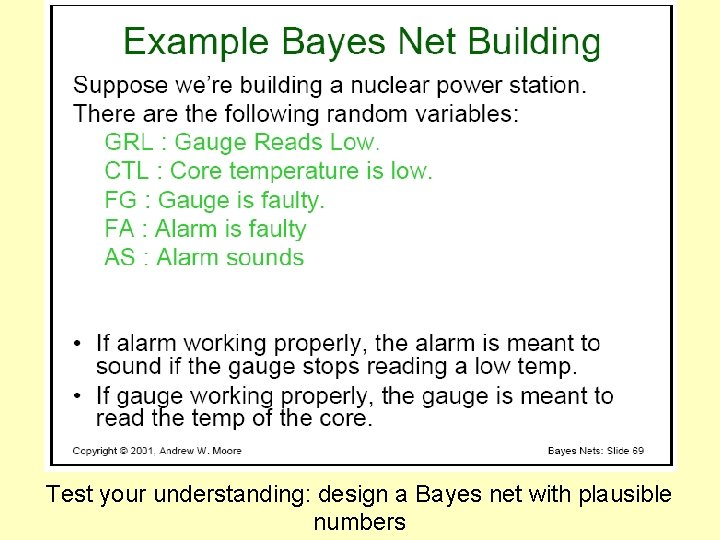Test your understanding: design a Bayes net with plausible numbers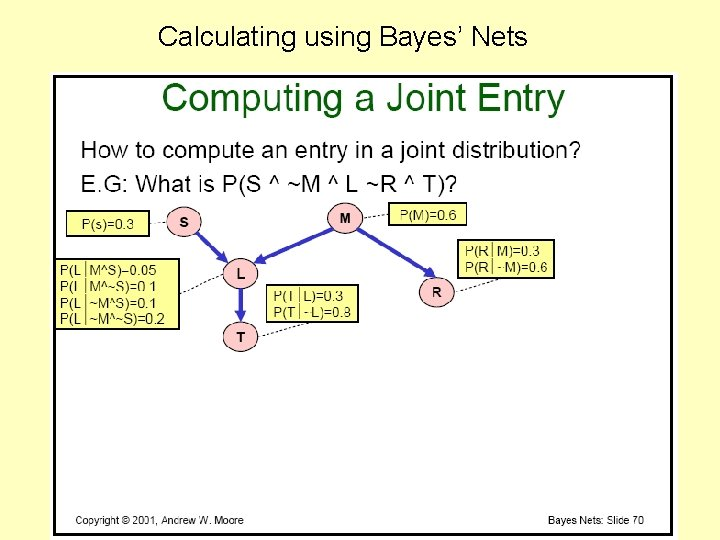Calculating using Bayes’ Nets